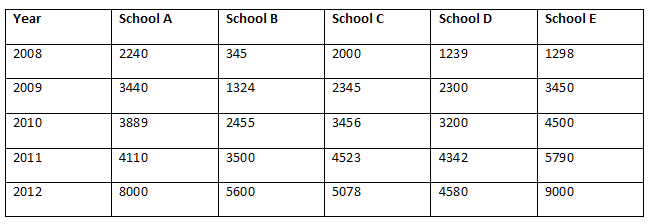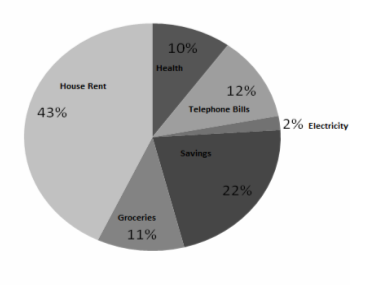# IBPS Quantitative Aptitude model test paper - 1

Home > Aptitude test > Quantitative model test - 1

## IBPS Quantitative Aptitude model test paper - 1

Directions (Q. 1-5 ) What approximate value should come in place of the question mark (?) in the following questions?

Q1. 8433.733 ÷ 54.102 ÷ 5.55 = ?

A. 27
B. 28
C. 25
D. 20

Q2. 3410648 = ?

A. 31
B. 23
C. 22
D. 32

Q3. 27.5% of 654 ÷ 22% of 67 = ?

A. 12
B. 14
C. 10
D. 18

Q4. (545.55 ÷ 11) ÷ 3.14 = ?

A. 15
B. 25
C. 31
D. 13

Q5. 43 * 23.34567 * ? = 12345.12

A. 13
B. 24
C. 9
D. 23

Directions (Q. 6-8) Fill the following Series

Q6. 65 128 ? 350 521 .

A. 169
B. 225
C. 221
D. 216

Q7. 122 146 172 ? 230 .

A. 198
B. 216
C. 196
D. 200

Q8. 25 100 225 400 ?

A. 500
B. 600
C. 575
D. 600

Directions (Q.9 -11) In the following series only one number is wrong. Find out the wrong number.

Q9. 1 27 127 343 729

A. 127
B. 1
C. 343
D. 27

Q10. 25 27 225 343 625

A. 25
B. 225
C. 625
D. None of these

Q11. 1 1 4 8 9 25 16 64

A. 1
B. 16
C. 25
D. 9

Direction (Q. 12 - 16): Study the following table carefully to answer the questions that follow:

Total number of students studying in Various Schools over the yearsQ12. What is the average number of students studying in the School C over the given years excluding the year 2010?

A. 3480.4
B. 13946
C. 17402
D. 3486.5
E. None of these

Q13. The number of students studying in school B in the year 2009 is approximately what percent of the total number of students studying in various schools in that year?

A. 19
B. 11
C. 9
D. 24
E. 33

Q14. What is the approximate ratio of the number of students studying in school B to the number of students studying in school E in the year 2008?

A. 1 : 4
B. 2: 3
C. 4: 2
D. 4:3
E. 4 :1

Q15. What is the difference between the average number of students studying in school B over the given years and the average number of students studying in school E over the given

years?

A. 362.8
B. 487.4
C. 2162.8
D. 348.2
E. None of these

Q16. What is the difference between the total number of students studying in school A over the given years and the average number of students studying in the school D over the given

years ?

A. 6000
B. 6400
C. 6018
D. 5800
E. None of these

Directions (Q. 17-21) Study the Pie-Chart carefully to answer the questions that follow :

Percentage wise break-up of Spending Pattern of John in a Month. Total Amount Spent in am Month = Rs. 30,000Q17. What is the amount spent by John on Groceries ?

A. 3300
B. 3000
C. 3600
D. 6600
E. None of these

Q18. What is the total amount spent by John on Telephone Bills, Health and Electricity together?

A. 7000
B. 7204
C. 6600
D. 5780
E. None of these

Q19. What is the approximately ratio of the amount spent by John on Groceries to the amount spent on House Rent?

A. 1:4
B. 1:2
C. 3:2
D. 2:3
E. None of these.

Q20. Amount invested by John on Saving Forms what percent of the amount spent on Health?

A. 1:3
B. 21:17
C. 33: 21
D. 3:1
E. None of these

Q21. Total amount spent by the family on Telephone Bills, Groceries and Electricity together forms what percent of the amount spent on House Rent?

A. 40
B. 58.13
C. 34.64
D.12.50
E. None of these

Q22. The product of two consecutive odd numbers is 4623. Which is the smaller of the two numbers?

A. 71
B. 73
C. 67
D. 57
E. None of these

Q23. If the numerator of a fraction is increased by 200% and denominator is increased by 400%, the resultant fraction is 12/18. What was the original fraction?

A. 10/9
B. 19/10
C. 18/12
D. 1/5
E. None of these

Q24. 45 men can do a piece of work in 20 days. How many men would be required to do the same work in 30 days ?

A. 40
B. 25
C. 28
D. 30
E. 32

Q25. Which number should replace both the question marks in the following equation?

? / 125 = 72 / ?

A. 96
B. 60
C. 2
D. 3
E. None of these

#### Discussion

• RE: IBPS Quantitative Aptitude model test paper - 1 -rajneesh (03/02/19)
• question 8 ans is 625
• RE: IBPS Quantitative Aptitude model test paper - 1 -SHASHI (03/10/18)
• sorry question no 8...
• RE: IBPS Quantitative Aptitude model test paper - 1 -SHASHI (03/10/18)
• CAN ANY ONE EXPLAIN 6 QUESTION..